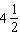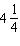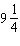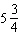Name:    Math 10W Unit 3 Practice Test #2

Multiple Choice
Identify the choice that best completes the statement or answers the question.

1.

What is the perimeter of a square garden with a side length of 3.2 m?
 a. 12.8 m c. 10.2 m b. 9.2 m d. 6.4 m

2.

How much wood do you need to form a triangular garden frame if one side of the frame has a length of 11 ft, and the other two sides are 2 feet longer than the first side?
 a. 36 ft c. 35 ft b. 33 ft d. 37 ft

3.

How many yards is 9 mi?
 a. 14 500 yards c. 16 040 yards b. 15 840 yards d. 12 672 yards

4.

What is the diameter of a circular window with a circumference of 1.35 m?
 a. 0.21 m c. 0.04 m b. 0.86 m d. 0.43 m

5.

Surface area is measured in which type of units?
 a. zero units c. linear units b. square units d. cube units

6.

The exterior of a lamp post is to be painted.  The lamp post is a 14-foot tall cylinder with a diameter of 18 inches. Since the base is on the ground and the top is covered by a floodlight, you will not need to paint these pieces. What is the area that needs to be painted?
 a. 113 in2 c. 792 in2 b. 9500 in2 d. 10009 in2

7.

Which of these shapes has the largest surface area?
• a cylinder with a height ofinches and a radius ofinches; or
• a rectangular prism with a length of 5 inches, a width ofinches, and a height of 4 inches; or
• a cone with a slanted side ofinches and a radius ofinches; or
• a square-based pyramid with a base length ofinches and a slant length ofinches.
 a. cylinder c. cone b. rectangular prism d. square-based pyramid

8.

Juan fills a 8 L bucket with a container that holds 2 pints. How many times will he have to fill up the container to fill the bucket?
 a. 8 times c. 17 times b. 9 times d. 10 times

9.

A garden has an area of 24 yd2. It is covered in topsoil that is 3 in deep. What is the volume of topsoil used, in yd3?
 a. 72 yd3 c. 6 yd3 b. 2 yd3 d. 24 yd3

10.

Which of the following has the largest volume?
 a. 30.5 quarts c. 87.5 pints b. 34 litres d. 10 US gallons

1.

Charlie drove for 3 hours at 80 km/h.

a)       How far did he travel in kilometres?

b)       How far did he travel in miles? Calculate the distance to the nearest mile.

2.

A company is selling decorative Christmas tree covers. After doing some research, the company finds that the average tree measures 6.5 feet high, has a diameter of 3.5 feet, and a slant height of 6.7 feet. How much material will be needed for the cover? Round to the nearest square foot.

3.

A garden measures 14 yd by 10 yd. In order to grow well, vegetables need about 0.8 m2 of space each. How many plants can you expect to grow in the garden?

4.

A rectangular prism measures 120 cm by 104 cm by 57 cm. Calculate its surface area in square inches. Round your answer to 2 decimal places.

5.

A can of diced tomatoes holds 5.5 fl oz. Your recipe calls for 225 mL of diced tomatoes.  Will you have enough tomatoes? (Hint: 1 fl oz = 30 mL.)